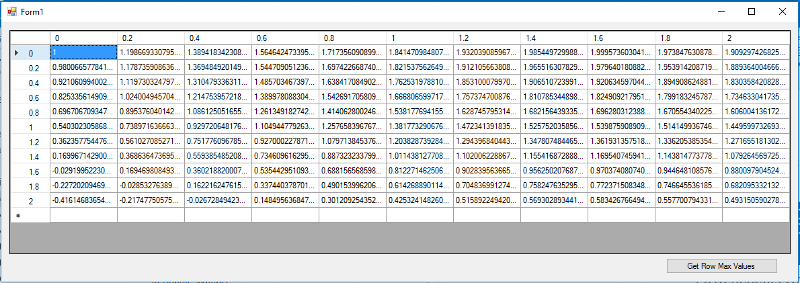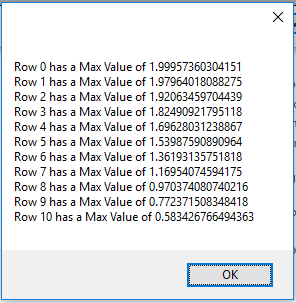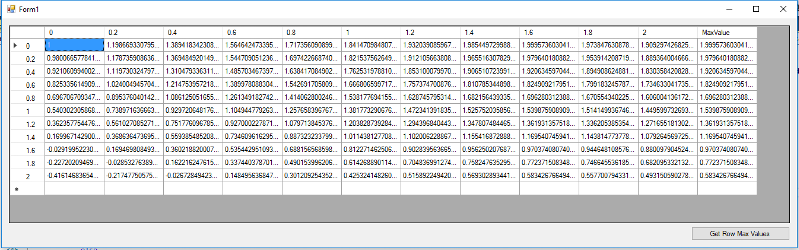# C# MAX VALUE DATAGRIDVIEW ROW

Hello,

how to find max value in datagridview (in row)

Thanks in advance!

My code:

``````public partial class Form1 : Form
{
public Form1()
{
InitializeComponent();
double x = 0;
double y = 0;
int index1 = 0;
int a = 0;
double[] array = new double;

while (x <= 2)
{
index1 = 0;
y = 0;
while (y <= 2)
{
array[index1] = Math.Cos(x) + Math.Sin(y);
y = y + 0.2;
index1++;
}

dataGridView1.Rows.Add(array, array, array, array, array, array, array, array, array, array, array, array);
dataGridView1.Rows[a].HeaderCell.Value = Convert.ToString(x);
a++;

x = x + 0.2;
}

}

private void dataGridView1_CellContentClick(object sender, DataGridViewCellEventArgs e)
{

}
}
``````
table.JPG
Asked:
###### Who is Participating?
I wear a lot of hats...

"The solutions and answers provided on Experts Exchange have been extremely helpful to me over the last few years. I wear a lot of hats - Developer, Database Administrator, Help Desk, etc., so I know a lot of things but not a lot about one thing. Experts Exchange gives me answers from people who do know a lot about one thing, in a easy to use platform." -Todd S.

Software Team LeadCommented:
a looping could be help here.

``````private void dataGridView1_CellContentClick(object sender, DataGridViewCellEventArgs e)
{
var maxValue = 0.0;
foreach (DataGridViewCell c in dataGridView1.Rows[e.RowIndex].Cells)
{
if (Convert.ToDouble(c.Value) > maxValue)
{
maxValue = Convert.ToDouble(c.Value);
}
}
MessageBox.Show(maxValue.ToString());
}
``````

Experts Exchange Solution brought to you by

Your issues matter to us.

Facing a tech roadblock? Get the help and guidance you need from experienced professionals who care. Ask your question anytime, anywhere, with no hassle.

Freelance programmer / ConsultantCommented:
Something like.

double max = 0.0;  //Caution - if numbers can be negative use a large negative number
foreach(DataGridViewCell c in dataGridView1.Rows)   //replace zero with the row index you require
{
if(max < Convert.ToDouble(c.Value)) max = Convert.ToDouble(c.Value);
}

max should now contain the max value
Author Commented:
Thanks for answers. I forgot to write that I need to get the max value of every row, how could I do that?

With this:
``````double max = 0.0;  //Caution - if numbers can be negative use a large negative number
foreach(DataGridViewCell c in dataGridView1.Rows)   //replace zero with the row index you require
{
if(max < Convert.ToDouble(c.Value)) max = Convert.ToDouble(c.Value);
}
``````
in foreach loop? When Rows.[] index will be element in foreach?
Freelance programmer / ConsultantCommented:
Yes a foreach loop over all of the rows will do it.  Note you would need something to store the results on a per row max basis, eg a List if you want the max in eac row, if you just wanted the largest value in the grid then the simple double to store the max is suitable.
Author Commented:
Can I export every row max value into a label ? Then I could check max values not in foreach but writing index by myself. And then 0 row will write max value to label1, first row to label2 and etc.
Senior .Net DeveloperCommented:
Just use a List<decimal>.

Eg:
``````List<Decimal> maxes = new List<Decimal>()
foreach (DataRow row in datagridview.Rows())
{
maxes.Add(FindMax(row));  // FindMax function will return the value of the max in a row
}
``````
Now maxes will be the max in the first row.

Note this is valid as long as the user doesn't sort.  If you need to handle sorting you could either repeat this function to regenerate the maxes OR you could build a dictionary to store a reference to the row.
Freelance programmer / ConsultantCommented:
>>Can I export every row max value into a label ?
Yes.  Note it isn't as simple as it sounds because of identifying the label for setting the text with the value of max.
Senior .Net DeveloperCommented:
Can you provide the code?
Freelance programmer / ConsultantCommented:
Instead of a label would for example a listbox be more suitable for the visual output?  If the list box isn't sorted you can rely on the ordering in the listbox being that of the rows.  Much simpler to code as well.

ps.  Doing it visually isn't efficient, it is better to use code unless you have a very specific reason for  displaying the max value in each row.

pps. You might want to consider a slight change, an extra column in the grid which is used to display the max value.  Slight changes to the code you have will prevent that being included in the checking for the max value.
Freelance programmer / ConsultantCommented:
foreach (DataGridViewRow row in datagridviews.Rows)
{

Code like that should work.
Author Commented:
I'm creating program for university and I need to find max values of every row and print it. So I think that label would be the best solution because I will create labels near every row and print max values of every row in it.
Freelance programmer / ConsultantCommented:
OK.  Have you got the foreach working yet?
DeveloperCommented:
Another implementation:

Form1.cs -
``````using System;
using System.Collections.Generic;
using System.Data;
using System.Linq;
using System.Windows.Forms;

namespace EE_Q29065398
{
public partial class Form1 : Form
{
public Form1()
{
InitializeComponent();
}

private void OnLoad(object sender, EventArgs e)
{
var tangents = (from x in Enumerable.Range(0, 11) select
(from y in Enumerable.Range(0, 11)
select new KeyValuePair<string, double>(\$"{y * .2}", Math.Cos(x * .2) + Math.Sin(y * .2))).ToDictionary(k => k.Key, v => v.Value));

var table = new DataTable("Tangents");
table.Columns.AddRange(tangents.First().Select(c => new DataColumn { ColumnName = c.Key, DataType = typeof(double) }).ToArray());
tangents.ToList().ForEach(r => table.Rows.Add(r.Select(c => c.Value).Cast<object>().ToArray()));
dataGridView1.DataSource = table;
}

private void OnDataBindingComplete(object sender, DataGridViewBindingCompleteEventArgs e)
{
if (sender is DataGridView)
{
var grid = sender as DataGridView;
if (grid.Rows.Count > 0)
{
foreach (var row in grid.Rows.Cast<DataGridViewRow>().Where(r => !r.IsNewRow))
row.HeaderCell.Value = grid.Columns[row.Index].HeaderCell.Value;
}
}
}

private void OnClick(object sender, EventArgs e)
{
var maxValues = (from row in dataGridView1.Rows.Cast<DataGridViewRow>().Where(r => !r.IsNewRow)
select \$"Row {row.Index} has a Max Value of {(row.DataBoundItem as DataRowView).Row.ItemArray.Cast<double>().Max()}");

MessageBox.Show(string.Join(Environment.NewLine, maxValues.ToArray()));
}
}
}
``````
Form1.Designer.cs -
``````namespace EE_Q29065398
{
partial class Form1
{
/// <summary>
/// Required designer variable.
/// </summary>
private System.ComponentModel.IContainer components = null;

/// <summary>
/// Clean up any resources being used.
/// </summary>
/// <param name="disposing">true if managed resources should be disposed; otherwise, false.</param>
protected override void Dispose(bool disposing)
{
if (disposing && (components != null))
{
components.Dispose();
}
base.Dispose(disposing);
}

#region Windows Form Designer generated code

/// <summary>
/// Required method for Designer support - do not modify
/// the contents of this method with the code editor.
/// </summary>
private void InitializeComponent()
{
this.dataGridView1 = new System.Windows.Forms.DataGridView();
this.button1 = new System.Windows.Forms.Button();
((System.ComponentModel.ISupportInitialize)(this.dataGridView1)).BeginInit();
this.SuspendLayout();
//
// dataGridView1
//
this.dataGridView1.ColumnHeadersHeightSizeMode = System.Windows.Forms.DataGridViewColumnHeadersHeightSizeMode.AutoSize;
this.dataGridView1.Location = new System.Drawing.Point(12, 12);
this.dataGridView1.Name = "dataGridView1";
this.dataGridView1.RowHeadersWidth = 60;
this.dataGridView1.Size = new System.Drawing.Size(1162, 333);
this.dataGridView1.TabIndex = 0;
this.dataGridView1.DataBindingComplete += new System.Windows.Forms.DataGridViewBindingCompleteEventHandler(this.OnDataBindingComplete);
//
// button1
//
this.button1.Location = new System.Drawing.Point(989, 351);
this.button1.Name = "button1";
this.button1.Size = new System.Drawing.Size(166, 23);
this.button1.TabIndex = 1;
this.button1.Text = "Get Row Max Values";
this.button1.UseVisualStyleBackColor = true;
this.button1.Click += new System.EventHandler(this.OnClick);
//
// Form1
//
this.AutoScaleDimensions = new System.Drawing.SizeF(6F, 13F);
this.AutoScaleMode = System.Windows.Forms.AutoScaleMode.Font;
this.ClientSize = new System.Drawing.Size(1185, 386);
this.Controls.Add(this.button1);
this.Controls.Add(this.dataGridView1);
this.Name = "Form1";
this.Text = "Form1";
this.Load += new System.EventHandler(this.OnLoad);
((System.ComponentModel.ISupportInitialize)(this.dataGridView1)).EndInit();
this.ResumeLayout(false);

}

#endregion

private System.Windows.Forms.DataGridView dataGridView1;
private System.Windows.Forms.Button button1;
}
}
``````
Produces the following results --saige-
Author Commented:
`````` double cmax = 0.0;  //Caution - if numbers can be negative use a large negative number
foreach (DataGridViewCell c in dataGridView1.Rows.Cells)   //replace zero with the row index you require
{
if (cmax < Convert.ToDouble(c.Value))
{
cmax = Convert.ToDouble(c.Value);
label1.Text = "Max value: " + cmax;
}
}
``````

It works. Thanks!
Senior .Net DeveloperCommented:
You could also just create another column in the datagridview itself:

myDgv.Columns.Add("Max", typeof(decimal));

foreach (DataRow row in myDgv.Rows)
row["Max"] = FindMax(row);
Author Commented:
Also I think about for or foreach loop for getting index. Can I write index to label?
For example:
Now I have:
``````label1.Text = "Max value: " + cmax;
``````
Can I have:
``````label[some index? How to write it?].Text = "Max value: " + cmax;
``````
?
Freelance programmer / ConsultantCommented:
Precisely why I said that approach with a label wasn't so simple.

As you want it displayed I really would suggest implementing what I suggested earlier with an extra column in the grid to display the max.  It would appear right next to the other values in the row so pretty clear which row it referred to.
Senior .Net DeveloperCommented:
@Andy my apologies missed that you had already suggested that.
Freelance programmer / ConsultantCommented:
Thanks Kyle, I guessed you might have done so.  (Shows it probably is a good idea though if two thought of it).
Author Commented:
I don't know how to make exception for not to check the last column in foreach loop
Senior .Net DeveloperCommented:
``````    foreach (DataGridViewCell c in dataGridView1.Rows.Cells)   //replace zero with the row index you require
{
if (c.Name == "Max") // Max = name of column
continue; // skip this loop iteration

if (cmax < Convert.ToDouble(c.Value))
{
cmax = Convert.ToDouble(c.Value);
label1.Text = "Max value: " + cmax;
}
}
``````
Author Commented:
And how I can add text to single cell, not in all row ?
I tried:
``````double cmax = -5.0;

int indexx = 0;
foreach (DataGridViewCell c in dataGridView1.Rows[indexx].Cells)
{

if (c.Equals("MaxReiksme")) // Max = name of column
continue; // skip this loop iteration
if (cmax < Convert.ToDouble(c.Value))
{
cmax = Convert.ToDouble(c.Value);
//label1.Text = "Max value: " + cmax;
dataGridView1.Rows.Cells.Add(cmax);
indexx++;
}
}
``````

But it says that cannot convert double to System.Windows.Forms.DataGridViewCell
Freelance programmer / ConsultantCommented:
instead of
dataGridView1.Rows.Cells.Add(cmax);
does this work?
dataGridView1.Rows.Cells["MaxReiksme"].Value = cmax.ToString();
Author Commented:
No...
"System.ArgumentOutOfRangeException: 'Index was out of range. Must be non-negative and less than the size of the collection.
Parameter name: index' "
Freelance programmer / ConsultantCommented:
ps.  You use ...Rows...  do you not mean ...Rows[indexx].....
Author Commented:
I tried with 12 and with indexx, it shows the same result.
I tried this:
``````dataGridView1.Rows[indexx].Cells.Value = cmax.ToString();
``````
12 because it's column number or name: "Column12" because MaxReiksme is only a header name
Freelance programmer / ConsultantCommented:
The twelth cell has the index 11, zero based indexing.
Author Commented:
Thanks. My program writes wrong max size but I will solve it by myself. Thanks for help!
DeveloperCommented:
Here is an implementation that illustrates what Andy and Kyle have alluded to:
``````using System;
using System.Collections.Generic;
using System.Data;
using System.Linq;
using System.Windows.Forms;

namespace EE_Q29065398
{
public partial class Form1 : Form
{
public Form1()
{
InitializeComponent();
}

private void OnLoad(object sender, EventArgs e)
{
var tangents = (from x in Enumerable.Range(0, 11) select
(from y in Enumerable.Range(0, 11)
select new KeyValuePair<string, double>(\$"{y * .2}", Math.Cos(x * .2) + Math.Sin(y * .2))).ToDictionary(k => k.Key, v => v.Value).AddMaxColumn());

var table = new DataTable("Tangents");
table.Columns.AddRange(tangents.First().Select(c => new DataColumn { ColumnName = c.Key, DataType = typeof(double) }).ToArray());
tangents.ToList().ForEach(r => table.Rows.Add(r.Select(c => c.Value).Cast<object>().ToArray()));
dataGridView1.DataSource = table;
}

private void OnDataBindingComplete(object sender, DataGridViewBindingCompleteEventArgs e)
{
if (sender is DataGridView)
{
var grid = sender as DataGridView;
if (grid.Rows.Count > 0)
{
foreach (var row in grid.Rows.Cast<DataGridViewRow>().Where(r => !r.IsNewRow))
row.HeaderCell.Value = grid.Columns[row.Index].HeaderCell.Value;
}
}
}

private void OnClick(object sender, EventArgs e)
{
var maxValues = (from row in dataGridView1.Rows.Cast<DataGridViewRow>().Where(r => !r.IsNewRow)
select \$"Row {row.Index} has a Max Value of {(row.DataBoundItem as DataRowView).Row.ItemArray.Take(10).Cast<double>().Max()}");

MessageBox.Show(string.Join(Environment.NewLine, maxValues.ToArray()));
}
}

static class Extensions
{
public static Dictionary<string, V> AddMaxColumn<V>(this Dictionary<string, V> source) where V : IComparable
{
source.Add("MaxValue", source.Aggregate((l, r) => l.Value.CompareTo(r.Value) > 0 ? l : r).Value);
return source;
}
}
}
``````
Which produces the following output -Freelance programmer / ConsultantCommented:
An example using the original suggestion method to obtain the max value:

``````        private void Form1_Load(object sender, EventArgs e)
{
Random r = new Random();
this.dataGridView1.Rows.Add(new object[] { r.Next(1000) / 100.0, r.Next(1000) / 100.0, r.Next(1000) / 100.0, r.Next(1000) / 100.0, r.Next(1000) / 100.0, r.Next(1000) / 100.0 });
this.dataGridView1.Rows.Add(new object[] { r.Next(1000) / 100.0, r.Next(1000) / 100.0, r.Next(1000) / 100.0, r.Next(1000) / 100.0, r.Next(1000) / 100.0, r.Next(1000) / 100.0 });
this.dataGridView1.Rows.Add(new object[] { r.Next(1000) / 100.0, r.Next(1000) / 100.0, r.Next(1000) / 100.0, r.Next(1000) / 100.0, r.Next(1000) / 100.0, r.Next(1000) / 100.0 });
this.dataGridView1.Rows.Add(new object[] { r.Next(1000) / 100.0, r.Next(1000) / 100.0, r.Next(1000) / 100.0, r.Next(1000) / 100.0, r.Next(1000) / 100.0, r.Next(1000) / 100.0 });
this.dataGridView1.Rows.Add(new object[] { r.Next(1000) / 100.0, r.Next(1000) / 100.0, r.Next(1000) / 100.0, r.Next(1000) / 100.0, r.Next(1000) / 100.0, r.Next(1000) / 100.0 });
this.dataGridView1.Rows.Add(new object[] { r.Next(1000) / 100.0, r.Next(1000) / 100.0, r.Next(1000) / 100.0, r.Next(1000) / 100.0, r.Next(1000) / 100.0, r.Next(1000) / 100.0 });
}

private void button1_Click(object sender, EventArgs e)
{
foreach (DataGridViewRow r in this.dataGridView1.Rows)
{
double max = 0.0;
for(int i = 0; i < 6; i++)
{
if (max < Convert.ToDouble(r.Cells[i].Value))
max = Convert.ToDouble(r.Cells[i].Value);
}
r.Cells.Value = max.ToString();
}
}
``````

andafter the button click to find the max value in the row###### It's more than this solution.Get answers and train to solve all your tech problems - anytime, anywhere.Try it for free Edge Out The Competitionfor your dream job with proven skills and certifications.Get started today Stand Outas the employee with proven skills.Start learning today for free Move Your Career Forwardwith certification training in the latest technologies.Start your trial today
C#

From novice to tech pro — start learning today.